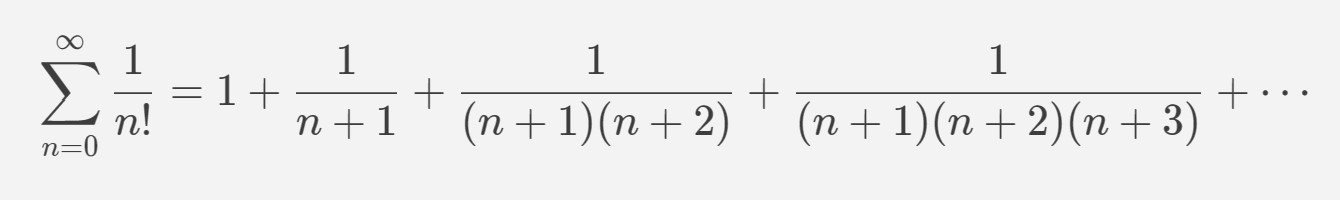# .Exp()

The `Math.Exp()` method returns the value of raising `e` to the power of a given number.

`e` stands for Euler’s number, an irrational number, with an approximate constant value of 2.71828. It provides a constant base to the natural logarithm or log and can be understood as a limit of a given progression.Consequently, the `Math.Exp()` method is the inverse of the `Math.Log()` method.

## Syntax

``````Math.Exp(double n);
``````

The method takes only one parameter; the variable `n` (number) of type `double`. The function will return a positive value of type `double` unless the value passed is one of the following:

• If `n` is `0`, the function will return `1`.
• If `n` is `PositiveInfinity`, the function will return `PositiveInfinity`.
• If `n` is `NegativeInfinity`, the function will return `0`.
• If `n` is `NaN`, the function will return `NaN`.

## Example

The following example applies the `Math.Exp()` method for six different values. The return values are printed with the `Console.WriteLine()` method.

```using System;
namespace MathExpMethod{  public class Example  {    public static void Main(string[] args)    {      double firstCase = Math.Exp(1);      double secondCase = Math.Exp(-1);      double thirdCase = Math.Exp(0);      double fourthCase = Math.Exp(double.PositiveInfinity);      double fifthCase = Math.Exp(double.NegativeInfinity);      double sixthCase = Math.Exp(double.NaN);
Console.WriteLine(firstCase);      Console.WriteLine(secondCase);      Console.WriteLine(thirdCase);      Console.WriteLine(fourthCase);      Console.WriteLine(fifthCase);      Console.WriteLine(sixthCase);    }  }}
```

This example results in the following output:

```2.718281828459050.3678794411714421Infinity0NaN
```

## Codebyte Example

The following example is runnable and uses the `Math.Exp()` method to return the value of raising `e` to the power of the given `double` type `number`:

`Favicon IconCodeOutputLoading...`